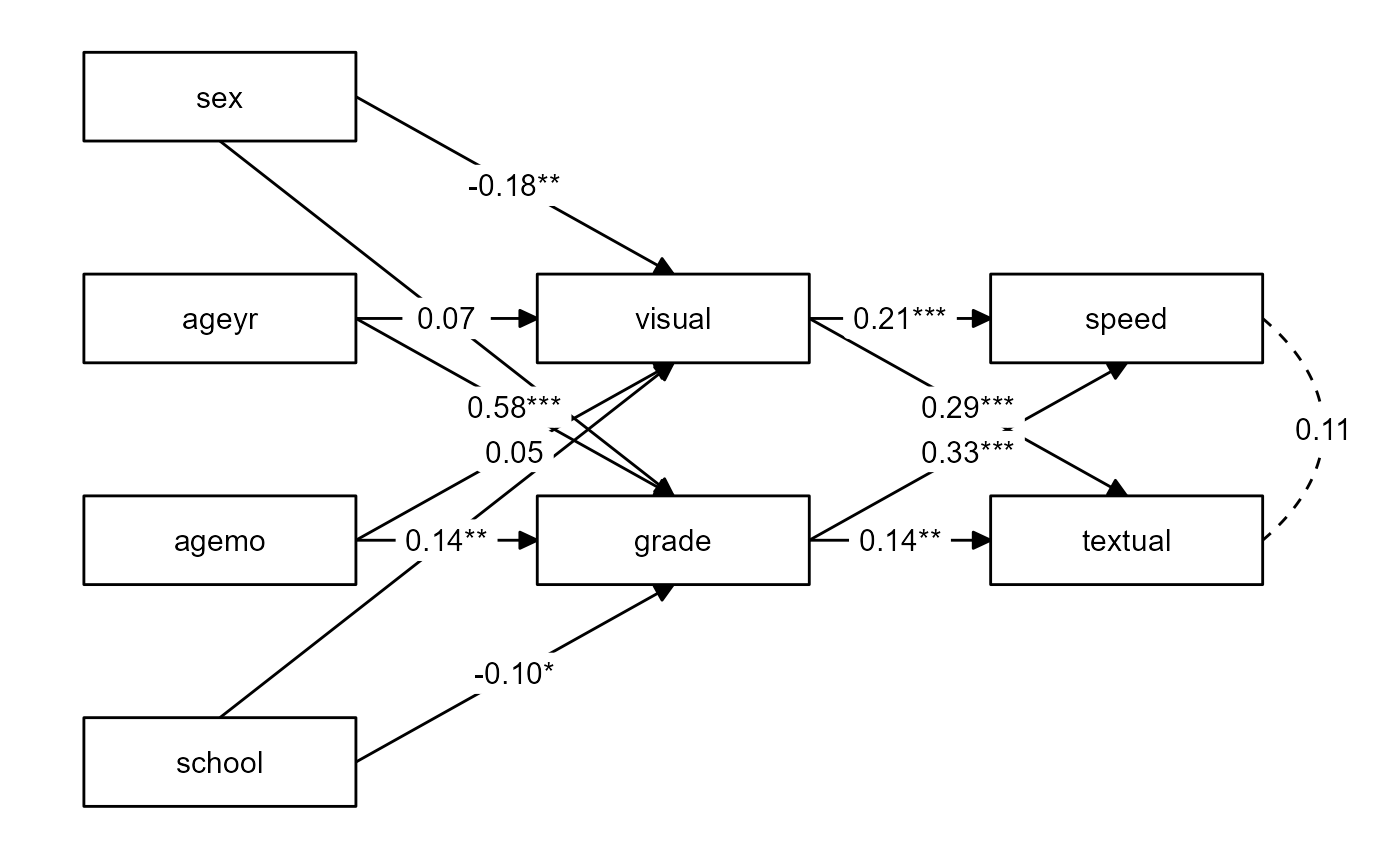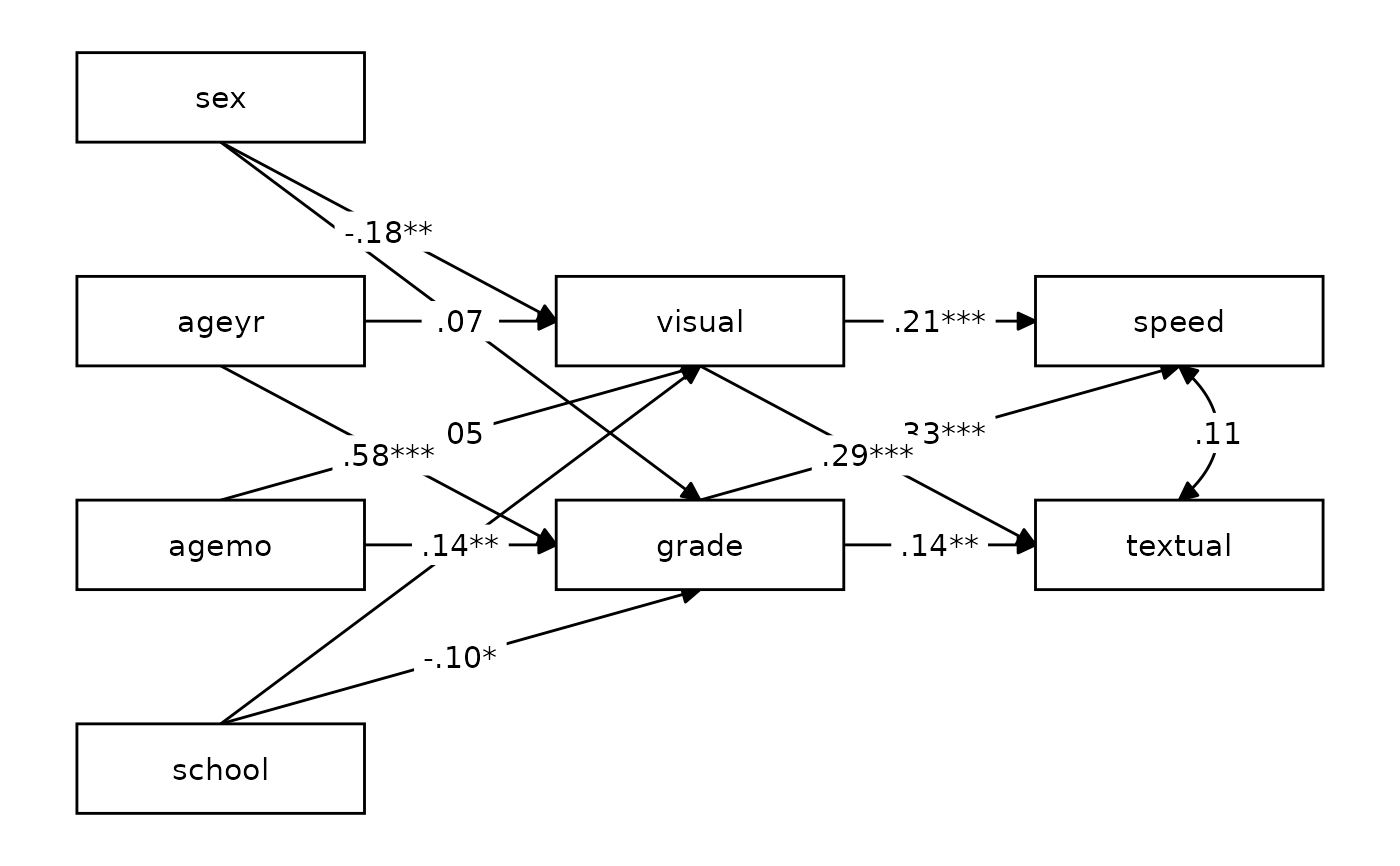Make a quick and decent-looking tidySEM plot.

## Usage

nice_tidySEM(
fit,
layout = NULL,
hide_nonsig_edges = FALSE,
hide_var = TRUE,
hide_cov = FALSE,
hide_mean = TRUE,
est_std = TRUE,
label,
label_location = NULL,
reduce_items = NULL,
plot = TRUE,
...
)

## Arguments

fit

SEM or CFA model fit to plot.

layout

A matrix (or data.frame) that describes the structure; see tidySEM::get_layout. If a named list is provided, with names "IV" (independent variables), "M" (mediator), and "DV" (dependent variables), nice_tidySEM attempts to write the layout matrix automatically.

hide_nonsig_edges

Logical, hides non-significant edges. Defaults to FALSE.

hide_var

Logical, hides variances. Defaults to TRUE.

hide_cov

Logical, hides co-variances. Defaults to FALSE.

hide_mean

Logical, hides means/node labels. Defaults to TRUE.

est_std

Logical, whether to use the standardized coefficients. Defaults to TRUE.

label

Labels to be used on the plot. As elsewhere in lavaanExtra, it is provided as a named list with format (colname = "label").

label_location

Location of label along the path, as a percentage (defaults to middle, 0.5).

reduce_items

A numeric vector of length 1 (x) or 2 (x & y) defining how much space to trim from the nodes (boxes) of the items defining the latent variables. Can be provided either as reduce_items = 0.4 (will only affect horizontal space, x), or reduce_items = c(x = 0.4, y = 0.2) (will affect both horizontal x and vertical y).

plot

Logical, whether to plot the result (default). If FALSE, returns the tidy_sem object, which can be further edited as needed.

...

Arguments to be passed to tidySEM::prepare_graph.

## Value

A tidySEM plot, of class ggplot, representing the specified lavaan model.

## Illustrations## Examples

# Calculate scale averages
library(lavaan)
data <- HolzingerSwineford1939
data$visual <- rowMeans(data[paste0("x", 1:3)]) data$textual <- rowMeans(data[paste0("x", 4:6)])
data\$speed <- rowMeans(data[paste0("x", 7:9)])

# Define our variables
IV <- c("sex", "ageyr", "agemo", "school")
DV <- c("speed", "textual")

# Define our lavaan lists
mediation <- list(speed = M, textual = M, visual = IV, grade = IV)

# Define indirect object
structure <- list(IV = IV, M = M, DV = DV)

# Write the model, and check it
model <- write_lavaan(mediation, indirect = structure, label = TRUE)
cat(model)
#> ##################################################
#> # [-----------Mediations (named paths)-----------]
#>
#> visual ~ sex_visual*sex + ageyr_visual*ageyr + agemo_visual*agemo + school_visual*school
#>
#> ##################################################
#> # [--------Mediations (indirect effects)---------]
#>
#> sex_visual_speed := sex_visual * visual_speed
#> sex_visual_textual := sex_visual * visual_textual
#> ageyr_visual_speed := ageyr_visual * visual_speed
#> ageyr_visual_textual := ageyr_visual * visual_textual
#> agemo_visual_speed := agemo_visual * visual_speed
#> agemo_visual_textual := agemo_visual * visual_textual
#> school_visual_speed := school_visual * visual_speed
#> school_visual_textual := school_visual * visual_textual